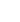15min:
OPTICAL ELECTRON PARAMETER SEMICONDUCTING NANOSTRUCTURES.

N. P. NETESOVA, M. V. Lomonosov Moscow State University, Physics Faculty, 119992, Moscow, Sparrow Hills, Russia.

Problem: to not use the classical Kramers-Kronig integral transformation and to define all optical electron oscillation parameters for any energy point from semiconducting nanostructure experimental reflection spectra\footnote N.~P.~ Netesova, NGS12 Proceedings , Editors: J.~Kono, Jean Leotin, Toulouse, France,\textbf 2, 178-183, (3-7 July 2005). Within the untied oscillation model the calculation technique of all semiconducting heterostructure optical parameters by the intermediate functions (\hbar, \hbarn, \hbar) are the plasma, effective natural, radiant friction energies in eV, 2\hbar is the Planck constant) is presented. As an example the optical parameters of PbS, PbSe, PbTe and GaAs, GaP between 0 and 25 eV in any spectrum region are established. The consistent approximation approach of the reflectance factor R to real value is advanced. As a result, all heterostructure basic electron optical functions (\hbarp, \hbarpm, \hbarc, \hbarare the plasma, plasma maximum, effective natural, radiant friction energies,r,, nr, nare the real and imaginary components of the dielectricand refractive index n functions, accordingly, (r)max, (r) min, (\hbaris conductivity, (\hbar)· n=(c\hbar/2)·, where c is the light velocity,is absorption coefficient, L=Im (-1/) are electron lossis, equal imaginary component of the minus reciprocal dielectric function, \hbar· L=(\hbar)· Im (-1/) are effective electron lossis) calculated by the intermediate functions in any electron optical spectrum region. Then, for GaP experimental reflection spectra it is selected the point \hbar22=10.5625·10-4, the intermediate parameters are \hbar22=10.5625 ·10-4, \hbar2n2=9.03130933157· 10-4, \hbar22=1.875029665786·10-4, the basic parameters are \hbar2p2=19.5902684716 \linebreak·10-4, \hbar2c2=5.28479085993 ·10-4, \hbar22=0.79237637701·10-4 (eV)2. The R values calculated by electron parameters coincide with the experimental values R (\hbar) to within 10-6\div10-10 for 12 symbol computation. By presented method the nanostructure oscillation electron parameters are determined for device producing.